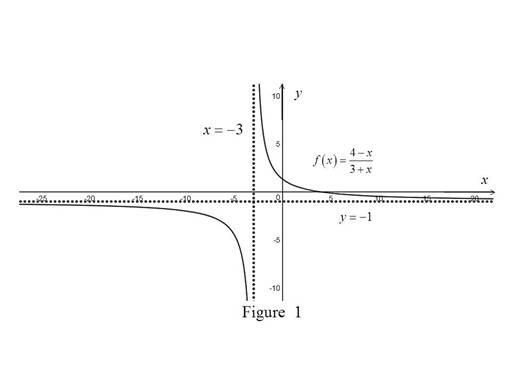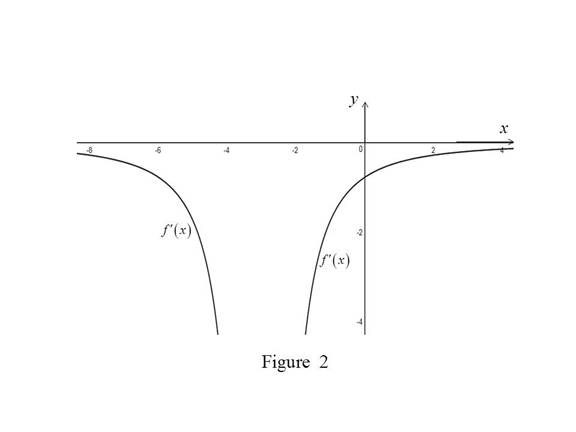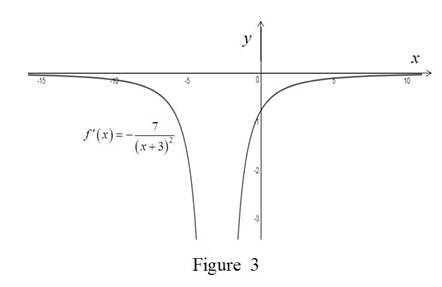# The asymptotes of the graph of f ( x ) = 4 − x 3 + x .### Single Variable Calculus: Concepts...

4th Edition
James Stewart
Publisher: Cengage Learning
ISBN: 9781337687805### Single Variable Calculus: Concepts...

4th Edition
James Stewart
Publisher: Cengage Learning
ISBN: 9781337687805

#### Solutions

Chapter 2, Problem 38RE

(a)

To determine

## To find: The asymptotes of the graph of f(x)=4−x3+x.

Expert Solution

The vertical asymptote is x=3 and horizontal asymptote is y=1.

### Explanation of Solution

Calculation:

Recall that the line y=a is horizontal asymptote if limx±f(x)=a.

f(x)=4x3+x

Take limit on the above.

limxf(x)=limx4x3+x=limx4x13x+1=413+1=1

Thus, the horizontal asymptote is y=1.

Recall that the line x=b is vertical asymptote if limxbf(x)=.

f(x)=4x3+x approaches infinity if 3+x approaches which implies x approaches 3.

limx3f(x)=limx3(4x)limx3(3+x)=7limx3(3+x)=

Thus, the vertical asymptote is x=3.

Graph:

Use the above information and trace the graph of f(x)=4x3+x as shown in Figure 1Observation:

x=3 is a discontinuous point of f.

(b)

To determine

Expert Solution

### Explanation of Solution

Calculation:

From the above graph in part a,

it is cleared that x=3 is a discontinuous point of f.

Both the two disjoint curves of f have negative slope.

Thus, the graph of f will have negative value.

For the curve present below the y-axis,

the value of the slope decreases as the curve moves from left to right

approaching the line x=3.

Thus, the graph of f in this part will be decreasing from left to right.

For the curve present above the y-axis,

the value of the slope increases as the curve moves from left to right

approaching the line y=1.

Thus, the graph of f in this part will be increasing from left to right.

Graph:

Use the information above and trace the graph of f as shown if Figure 2(c)

To determine

### To find: The derivative of f using the definition.

Expert Solution

The derivative is f(x)=7(x+3)2.

### Explanation of Solution

Formula used:

The derivative of a function f, denoted by f(x), is

f(x)=limh0f(x+h)f(x)h (1)

Calculation:

Obtain the derivative of the function f(x).

Compute f(x) by using the equation (1),

f(x)=limh0f(x+h)f(x)h=limh04(x+h)3+(x+h)4x3+xh

Simplify the numerator in the above expression

f(x)=limh0h(3+x)(4x)hh(3+x+h)(3+x)

Since the limit h approaches zero but not equal to zero, cancel the common term h from both the numerator and the denominator,

f(x)=limh0(3+x)(4x)(3+x+h)(3+x)=limh07(3+x+h)(3+x)=7(3+x+0)(3+x)=7(3+x)2

Thus, the value of the derivative is f(x)=7(x+3)2.

(d)

To determine

Expert Solution

### Explanation of Solution

Graph:

Use an online graphing calculator to sketch the graph of f(x)=7(x+3)2 as shown in Figure 3Observation:

From Figure 3, it has been observed that y=0 is a horizontal asymptote of f

Comparison:

The function in Figure 3 seems to be the same graph which was sketched in part b.

### Have a homework question?

Subscribe to bartleby learn! Ask subject matter experts 30 homework questions each month. Plus, you’ll have access to millions of step-by-step textbook answers!